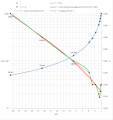# Li-ion cell charging module resistor selection?

#### Gian1

Joined Jun 10, 2020
4
Greetings. A vendor selling TP4056-based 5 V charging modules for Li-ion cells specified the following resistance values and resulting current for selecting the current-programming resistor:

 R (kΩ) I (mA) 30​ 50​ 20​ 70​ 10​ 130​ 5​ 250​ 4​ 300​ 3​ 400​ 2​ 580​ 1.66​ 690​ 1.5​ 780​ 1.33​ 900​ 1.2​ 1000​
On account of Ohm's law the range, median, and mean of the calculated voltage are, respectively, 1145.4 V - 1500 V; 1200 V; 1247.5 V. I have interpolated the values and fitted a cubic polynomial regression thus:The stock resistor resistance is 1.2 kΩ and, on the basis of its length and width of 1.6 mm × 0.8 mm, its power rating should be 1/16 W or thereabouts. With this resistor the resulting current is 1 A according to the TP4056 specifications.

Now, why does the calculated voltage have such high values and such a wide range? How should one calculate resistance values in order to get lower amperages, e.g. 4 mA? If the actual voltage across the current-programming resistor is 5 V or 4.2 V—which one is it?—and the current is 1 A, should not the resistor power rating be greater than or equal to 5 W or 4.2 W?

Thanks.

Last edited:

#### Gian1

Joined Jun 10, 2020
4
Had I read the TP4056 specifications I myself have linked instead of cursorily scanning it through I would have found out that the current-programming resistance formula is the following:

Rprog = (Vprog ⋅ 1200) / Icell

with Vprog = 1 V = current-programming resistor voltage; Rprog = current-programming resistor resistance; and Icell = charging current.

Moreover, the maximum current-programming resistor current is 1.2 mA, therefore it appears that the charging module stock resistor power rating is inadequate as it should be greater than or equal to 1.44 W.

#### Irving

Joined Jan 30, 2016
2,311
Moreover, the maximum current-programming resistor current is 1.2 mA, therefore it appears that the charging module stock resistor power rating is inadequate as it should be greater than or equal to 1.44 W.
Are you sure? Since Vprog = 1v the power dissipated in Rprog is always 1/Rprog Watts or 1 x Iprog W. Which is 1.2mW or less...

#### Gian1

Joined Jun 10, 2020
4
My mistake: I multiplied Rprog by Iprog instead of multiplying Vprog by Iprog. The required power rating for a 1.2 kΩ current-programming resistor is indeed 1.2 mW.

Last edited:

#### Gian1

Joined Jun 10, 2020
4
Would it be acceptable to use a potentiometer in place of the current-programming resistor?

#### Irving

Joined Jan 30, 2016
2,311
Yes, no reason not to, as long as you check charging current. You'll need a 50k multiturn pot, a normal pot is too coarse.

•Gian1